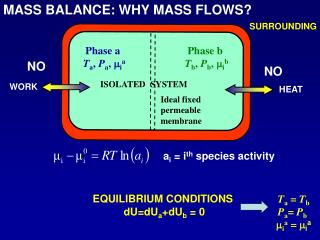DownloadDownload PresentationSURROUNDING

# SURROUNDING

Télécharger la présentation## SURROUNDING

- - - - - - - - - - - - - - - - - - - - - - - - - - - E N D - - - - - - - - - - - - - - - - - - - - - - - - - - -
##### Presentation Transcript

1. MASS BALANCE: WHY MASS FLOWS? SURROUNDING Phase a Ta, Pa, mia Phase b Tb, Pb, mib NO NO ISOLATED SYSTEM WORK HEAT Ideal fixed permeable membrane ai = ith species activity Ta = TbPa= Pb mia = mia EQUILIBRIUM CONDITIONS dU=dUa+dUb = 0

2. MASS BALANCE: EQ.CON. ALTERATION 1 Pa increase (>Pb) [Ta = Tb; mia = mib] Phase a T, Pa, mi Phase b T, Pb, mi MASS TRASPORT (CONVECTION) permeable membrane

3. mia>mib [Ta = Tb; Pa = Pb] 2 Phase a T, P, mia Phase b T, P, mib permeable membrane MASS TRASPORT (DIFFUSION) Mass transport represents a possible way the system has to get new equilibrium conditions once the original ones have been altered.

4. MASS BALANCE Z X Y DZ DX (X+DX, Y+ DY, Z+ DZ) G (X, Y, Z) DY (X, Y+ DY, Z) (X+DX, Y+ DY, Z)

5. MASS BALANCE: EXPRESSION DX DZ (X, Y, Z) G DY Ci = f(X, Y, Z, t)

6. DX DZ (X, Y, Z) G DY DIVIDING FOR DV

7. FLUXES EXPRESSIONS Ideal solution Diffusion Diffusion Diffusion Convection Convection Convection Bi = mobility of the diffusing components

8. Remembering that:

9. MASS BALANCE EQUATION FOR ith SPECIES where: Remembering the definition of the NABLA operator:

10. As: … the summation of ith mass balance over all the r components yields to the well known continuity equation:

11. MASS BALANCE: CYLINDRICAL COORDINATES Jir+dr Jir+dr Jiz Jiz+dz Jir Jir Jir Jiz Jiz+dz Jir+dr r dr dz TWO DIMENSIONS r z

12. Ci = f(z, r, t) r dr dz Dividing by 2prdrdz

13. Constant diffusion coefficient D Remembering the derivativedefinition

14. MOMENTUM BALANCE Incoming sand Escaping sand Sand containing vessel Sliding vessel motion direction Inclinate plane Friction Gravity NO Yes

15. DZ DX (X+DX, Y+ DY, Z+ DZ) (X, Y, Z) DY (X, Y+ DY, Z) (X+DX, Y+ DY, Z) Body forces: gravitational, electro-magnetic fields Surface forces: viscous drag and pressure

16. Surface forces gravitational field Incoming and exiting mass

17. ENERGY BALANCE Conduction Expansion Viscous heating